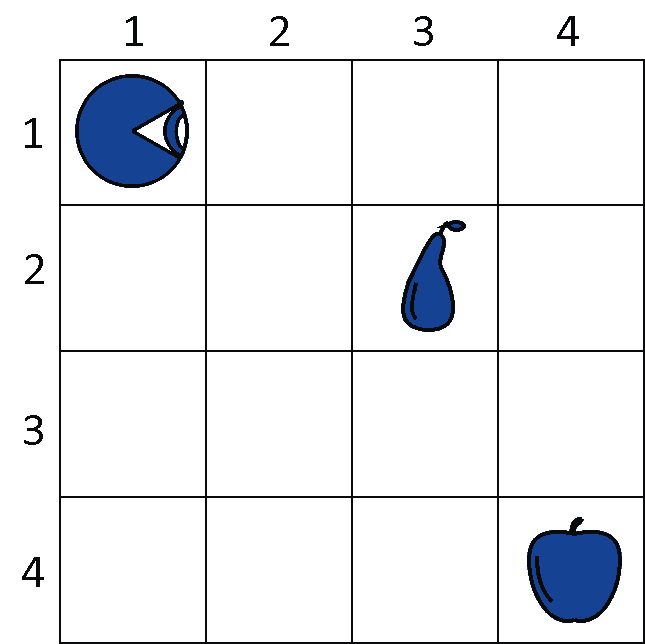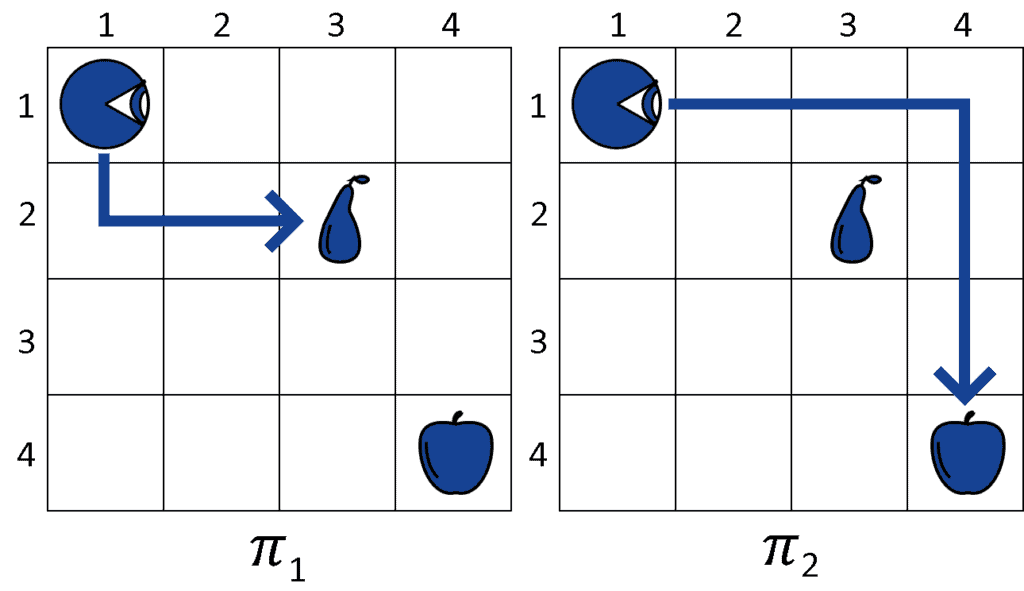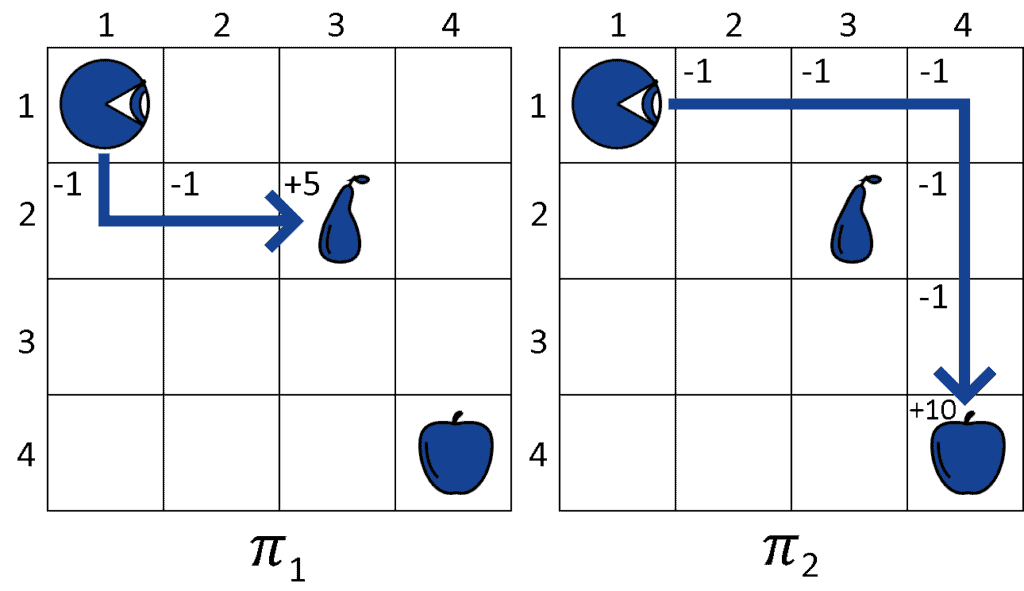## 1. Overview

In this tutorial, we’ll study the concept of policy for reinforcement learning.

At the end of this article, we’ll be familiar with the basic notions of reinforcement learning and its policy-based methods.

## 2. The Definition of a Policy

Reinforcement learning is a branch of machine learning dedicated to training agents to operate in an environment, in order to maximize their utility in the pursuit of some goals.

Its underlying idea, states Russel, is that intelligence is an emergent property of the interaction between an agent and its environment. This property guides the agent’s actions by orienting its choices in the conduct of some tasks.

We can say, analogously, that intelligence is the capacity of the agent to select the appropriate strategy in relation to its goals. Strategy, a teleologically-oriented subset of all possible behaviors, is here connected to the idea of “policy”.

A policy is, therefore, a strategy that an agent uses in pursuit of goals. The policy dictates the actions that the agent takes as a function of the agent’s state and the environment.

## 3. Mathematical Definition of a Policy

With formal terminology, we define a policyin terms of the Markov Decision Process to which it refers. A Markov Decision Process is a tuple of the form, structured as follows.

The first element is a setcontaining the internal states of the agent.  Together, all possible states span a so-called state space for the agent. In the case of the grid worlds for agent simulations,normally consists of the position of the agent on a board plus, if necessary, some parameters.

The second element is a setcontaining the actions of the agent. The actions correspond to the possible behaviors that the agent can take in relation to the environment. Together, the set of all actions spans the action space for that agent.

An action can also lead to a modification of the state of the agent. This is represented by the matrixcontaining the probability of transition from one state to another. Its elements,, contain the probabilitiesfor all possible actionsand pairs of states.

The fourth elementcomprises the reward function for the agent. It takes as input the state of the agent and outputs a real number that corresponds to the agent’s reward.

We can now formally define the policy, which we indicate with. A policycomprises the suggested actions that the agent should take for every possible state.

## 4. Example of a Policy in Reinforcement Learning

Let’s now see an example of policy in a practical scenario, to better understand how it works. In this example, an agent has to forage food from the environment in order to satisfy its hunger. It then receives rewards on the basis of the fruit it eats:The internal state of the agent corresponds to its location on the board, in this case,and. The action space, in this example, consists of four possible behaviors:. The probability matrixcontains all pairwise combinations of statesfor all actions in. It’s Bernoulli-distributed, and looks like this:The reward functionis defined in this manner. If it’s in an empty cell, the agent receives a negative reward of -1, to simulate the effect of hunger. If instead, the agent is in a cell with fruit, in this case,for the pear andfor the apple, it then receives a reward of +5 and +10, respectively.

The reward functionthus looks like this:

•••The simulation runs for an arbitrary finite number of time steps but terminates early if the agent reaches any fruit.

## 5. Evaluation of the Policies

The agent then considers two policiesand. If we simplify slightly the notation, we can indicate a policy as a sequence of actions starting from the state of the agent at:

1.2.The agent then has to select between the two policies. By computing the utility functionover them, the agent obtains:

••The evaluation of the policies suggests that the utility is maximized with, which then the agent chooses as its policy for this task.

## 6. Conclusions

In this article, we studied the concept of policy for reinforcement learning agents. We also studied one example of its application.

Inline FeedbacksNasim
6 months ago

Hello Gabriele,

I have this question that what is the difference between policy and heuristic and control in reinforcement learning?

Thanks,
NasimLoredana Crusoveanu
3 months ago